# High School Physics : Understanding Conservation of Energy

## Example Questions

2 Next →

### Example Question #11 : Understanding Conservation Of Energy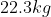child descends a slide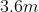high and reaches the bottom with a speed of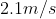.  How much thermal energy due to friction was generated in this process?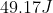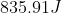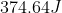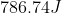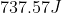Explanation:

We can use conservation of energy to solve this problem.  Let us consider the types of energy at the beginning and the end.  At the beginning the child has gravitational potential energy at the top of the slide.  When the child reaches the bottom, the child has both kinetic energy and thermal energy as some energy was converted to heat because of the friction on the slide.

The law of conservation of energy states that we can set the energy at the beginning equal to the energy at the end.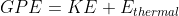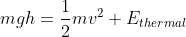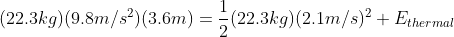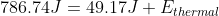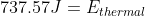Thisdifference between the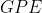at the top and the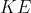at the bottom is the energy lost to friction.

### Example Question #12 : Understanding Conservation Of Energy

Conceptual

A man stands on a tall ladder of height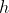. He leans over a little too far and falls off the ladder. If his mass is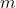, what is his velocity right before he hits the ground?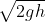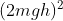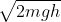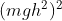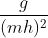Explanation:

For this problem, use the law of conservation of energy. This states that the total energy before the fall will equal the total energy after the fall. The initial kinetic energy will be zero, and the final potential energy will be zero; thus, the initial non-zero potential energy will be equal to the final non-zero kinetic energy.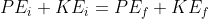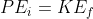From there, expand the equation to include the individual formulas for potential and kinetic energy calculation.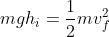Notice that the mass will cancel out from both sides.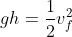Now we can solve for the final velocity in terms of the initial height.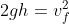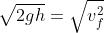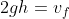### Example Question #13 : Understanding Conservation Of Energy

A runner arrives at the bottom of a hill. He runs up the hill with a constant acceleration until he reaches the top, then runs at a steady pace along the top of the hill. When are the kinetic and potential energies of this man at their greatest?

Potential energy is greatest at the top of the hill and kinetic energy is greatest at the bottom of the hill

Potential energy is greatest at the bottom of the hill and kinetic energy is greatest at the bottom of the hill

Potential energy is greatest at the top of the hill and kinetic energy remains constant

Potential energy is greatest at the bottom of the hill and kinetic energy is greatest at the top of the hill

Potential energy is greatest at the top of the hill and kinetic energy is greatest at the top of the hill

Potential energy is greatest at the top of the hill and kinetic energy is greatest at the top of the hill

Explanation:

To answer this question, we can address each type of energy separately. There is no conservation of energy in this problem; kinetic energy is not converted to potential energy as the man runs up the hill. Instead, he is accelerating, indicating an outside force that disallows conservation of energy.

First, we will find the maximum potential energy using the equation: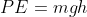The man's mass and the acceleration of gravity will remain constant. The only changing variable is height. When the height is greatest, the potential energy will be the greatest. We can conclude that the potential energy will thus be greatest at the top of the hill.

Now we will look at the equation for kinetic energy: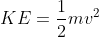The man's mass will remain constant, and the only changing variable will be the velocity. We are told that the man accelerates as he runs up the hill, indicating that his velocity is increasing. This tells us that he will reach a maximum velocity when he reaches the top of the hill, at which point he maintains a steady velocity along the top of the hill. Since kinetic energy is at a maximum when velocity is at a maximum, we can conclude that kinetic energy is greatest at the top of the hill.

### Example Question #14 : Understanding Conservation Of Energy

Two balls are thrown off a building with the same speed.  One is thrown straight up, and one at a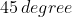angle.  Which statement is true if air resistance is ignored?

Both hit the ground with the same speed

Both a and b

Both hit the ground at the same time.

The one thrown at an angle hits the ground with a higher speed

The one thrown at an angle hits the ground with a lower speed

Both hit the ground with the same speed

Explanation:

We can use conservation of energy to analyze this problem.  When both balls are thrown off the building, they both have the same initial gravitational potential energy.  Additionally, since both balls are thrown with the same magnitude of the speed, they both have the same kinetic energy.  Energy is a scalar quantity and therefore does not have a direction.  Therefore it is independent of the path taken.

At the bottom, both balls will have converted all their potential energy and kinetic energy that they started with to kinetic energy at the bottom of the building.  Since both started with the same amount of total energy at the beginning, their kinetic energy at the end will also be the same.  Since both balls are assumed to have the same mass, their magnitude of their velocity (speed) will be the same as well.  However, their velocity will be in different directions because of the way that the ball was launched.

### Example Question #15 : Understanding Conservation Of Energy

A projectile is fired at an upward angle of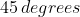from the top of a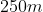cliff with a speed of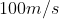.  What will be its speed when it strikes the ground below? (Use conservation of energy to solve this problem)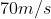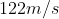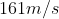Explanation:

Knowns: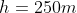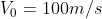Unknowns: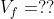The easiest way to solve this problem is by analyzing the energy of the object at different points during its path.  The first point to analyze is the moment it is launched from the top of the cliff.  Assuming that the ground is our zero point in reference, the projectile has both Gravitational Potential  Energy and Kinetic Energy when it is launched.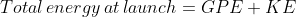At the end when the projectile lands on the ground, it no longer has gravitational potential energy.  It now is all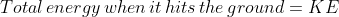We know that based on the law of conservation of energy, the total energy at the beginning must equal the total energy at the end.  Therefore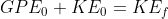Our equations for each are as follows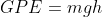We can substitute these equations in.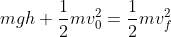Notice that mass is in every term so we can cancel it out.  That is why mass does not matter for this problem.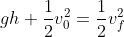Substitute in our values and solve for the final velocity.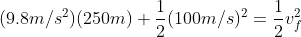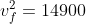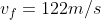### Example Question #16 : Understanding Conservation Of Energy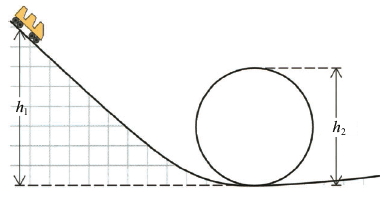Find the minimum initial height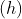of the roller coaster if the roller coaster is to complete the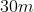diameter loop.

48.3m

50.8m

40.2m

12.1 m

37.5m

37.5m

Explanation:

First, we need to determine how fast the roller coaster must be going at the top of the loop to continue in a circular motion.  At the top of the loop, the only force acting on the car is gravity.  Therefore the gravitational force must be the cause of the centripetal motion.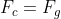We know that the force of gravity is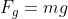And the centripetal force equation is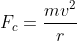We can set these two equations equal to each other.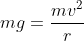Since mass is on both sides of the equation we can cancel it out.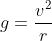We can rearrange and solve this equation for the velocity.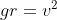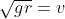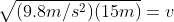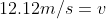We can now use the conservation of energy to determine the initial height of the roller coaster.  We know at the top of the roller coaster, there is only.  At the top of the loop of the coaster there is bothand.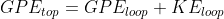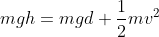Since mass is each factor, we can cancel it out.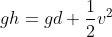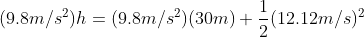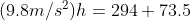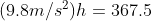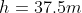The height of the coaster must start at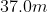.

2 Next →